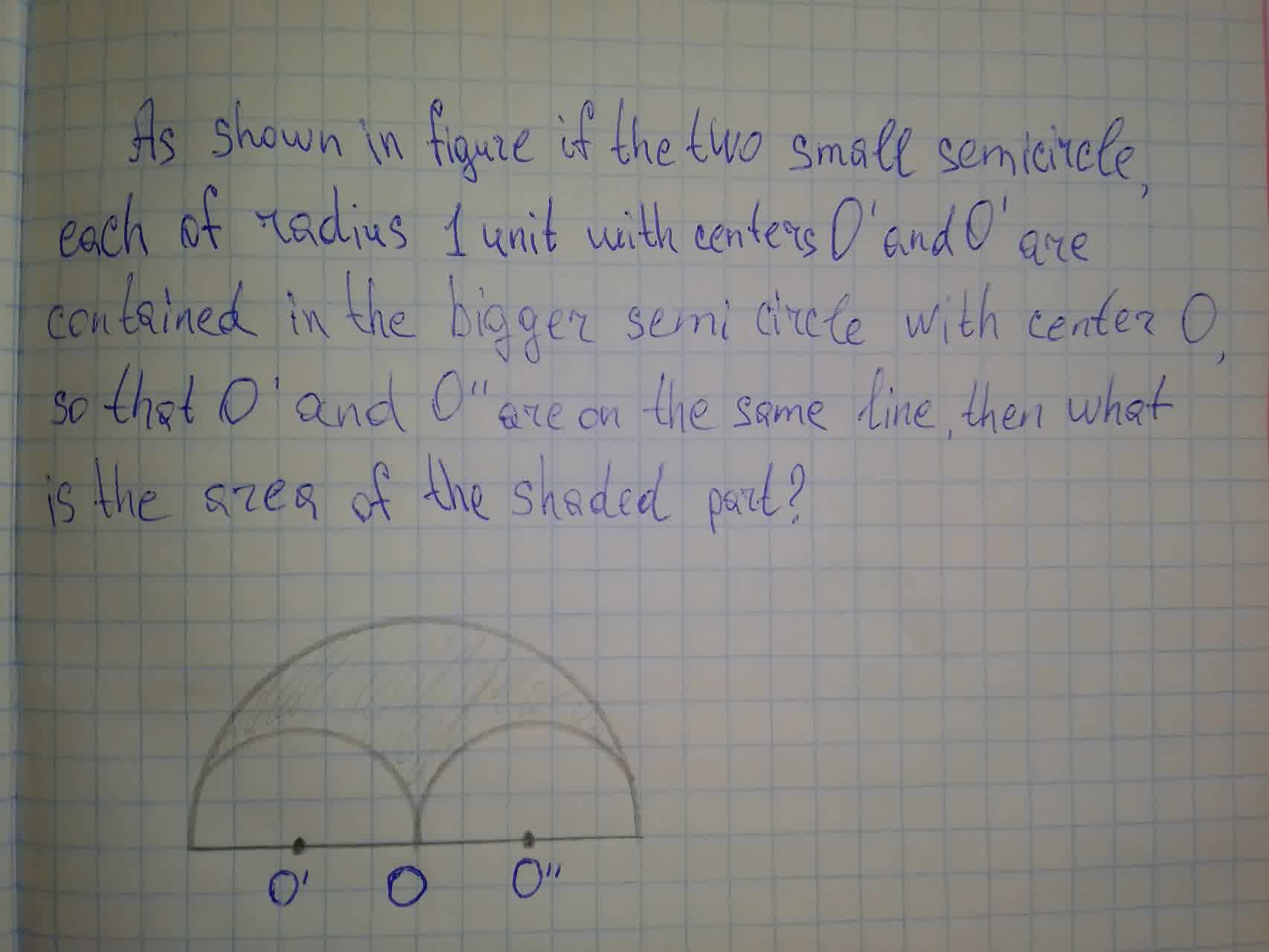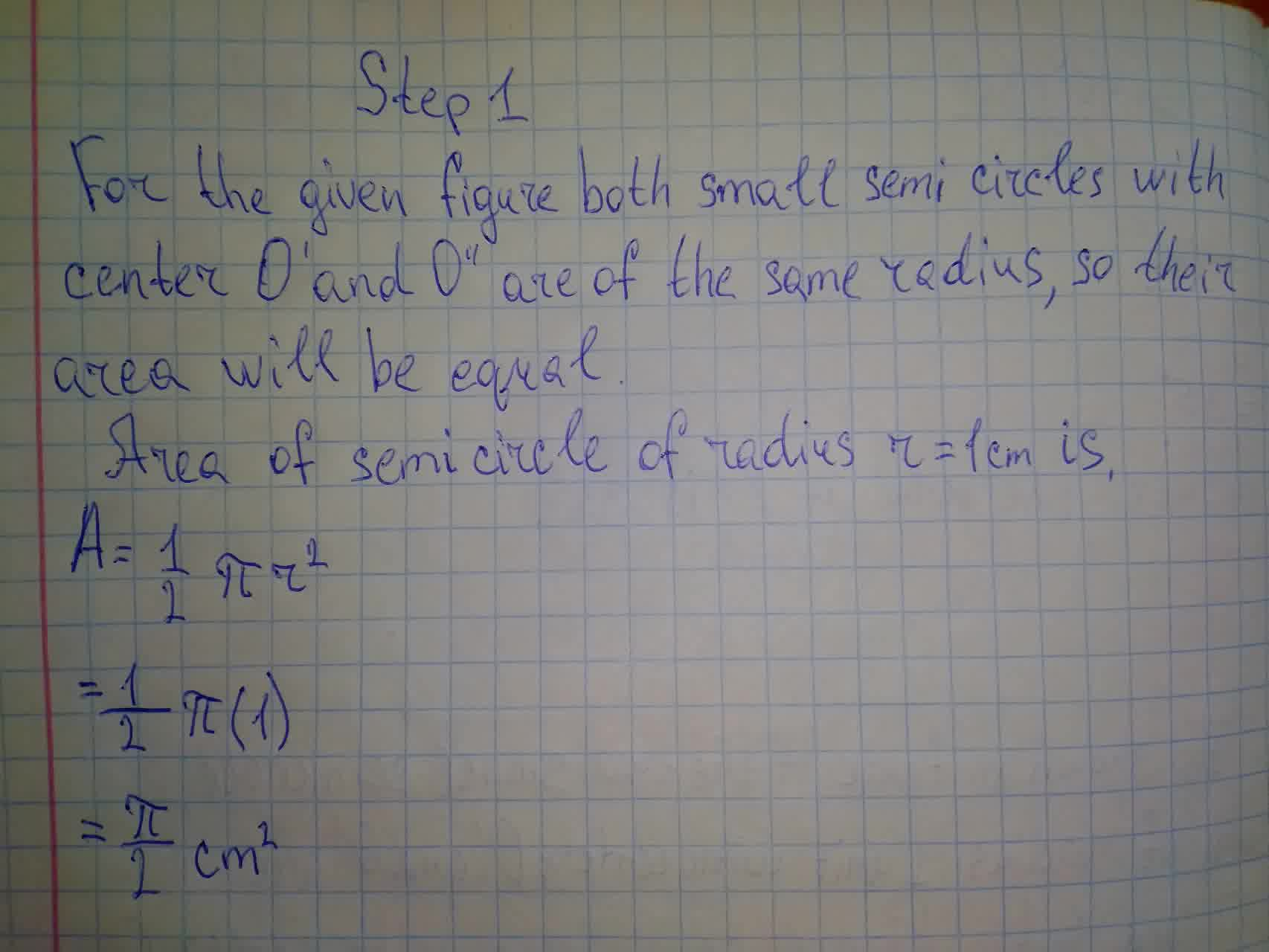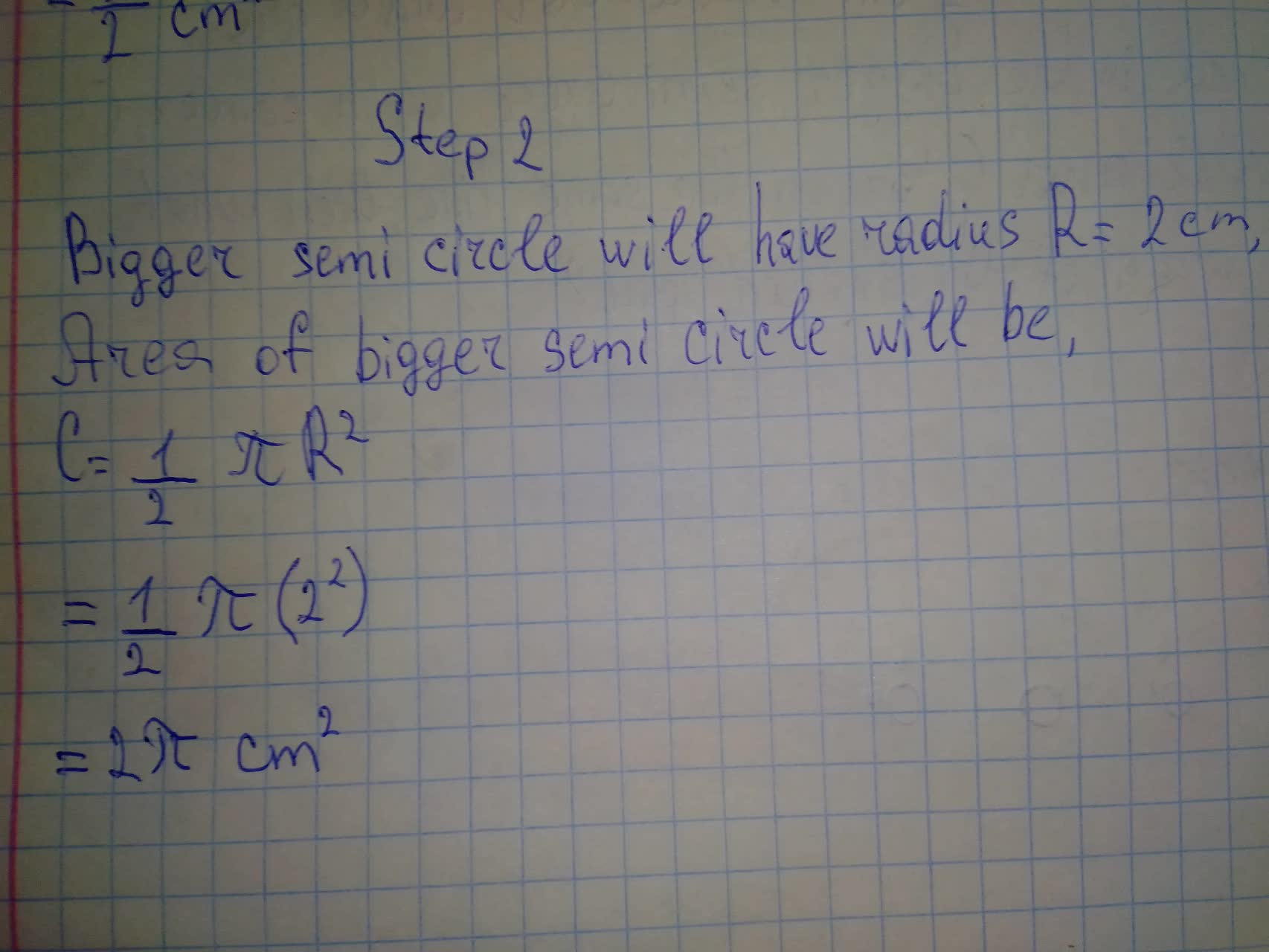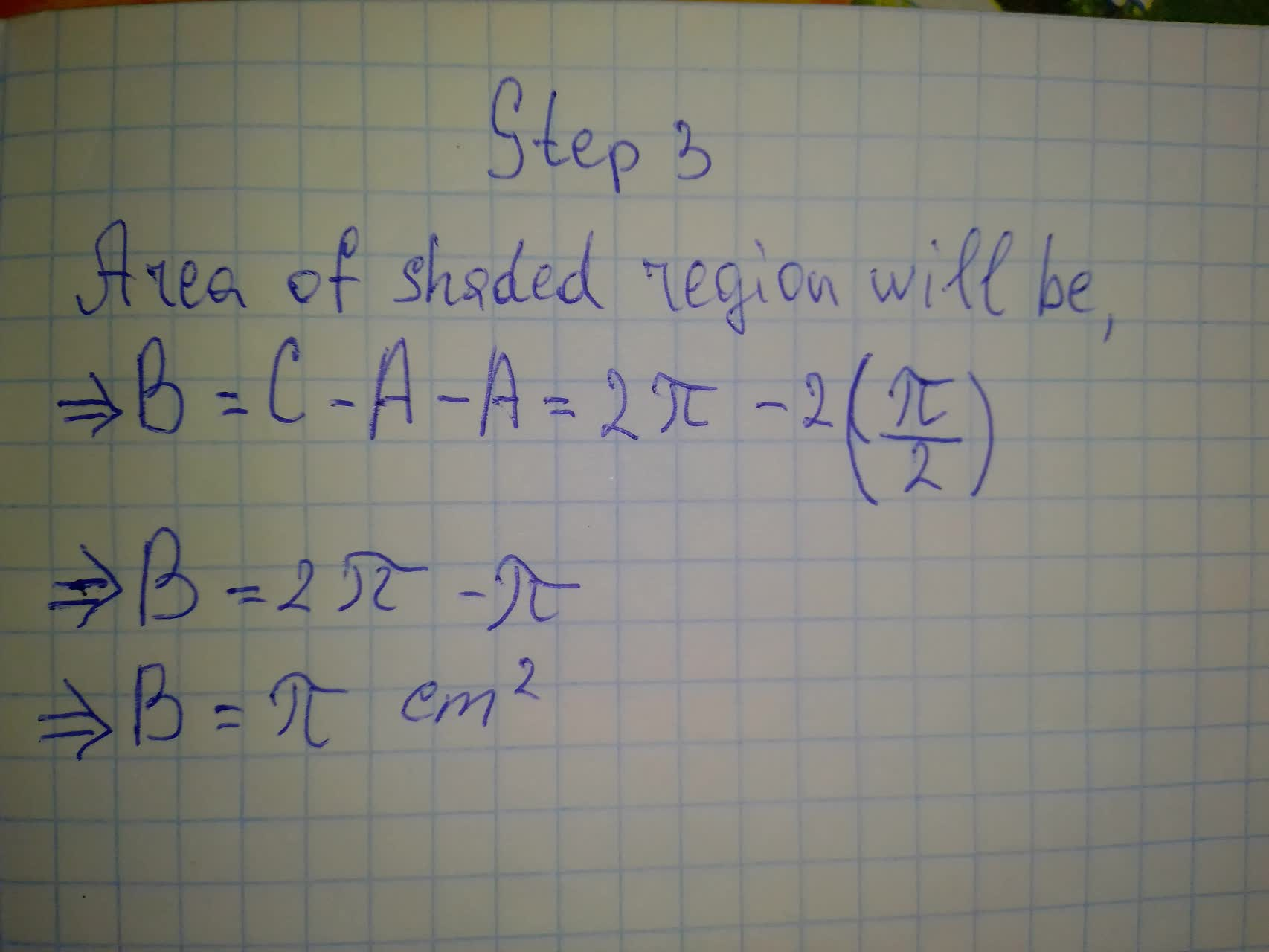Question# As shown in figure 5.111 to the right if the two small semicircle, each of radius 1 unit with centers O' and O' are contained in the bigger semi circle with center O

Circles
ANSWEREDAs shown in figure 5.111 to the right if the two small semicircle, each of radius 1 unit with centers O' and O' are contained in the bigger semi circle with center O, so that O' and O'' are on the same line, then what is the area of the shaded part?